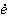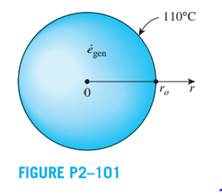Create an Account

Home / Questions / Consider a homogeneous spherical piece of radioactive material of radius

Consider a homogeneous spherical piece of radioactive material of radius ro = 0.04 m that is generating heat at a constant rate ofgen = 5 × 107 W/m3. The heat generated is dissipated to the environment steadily. The outer surface of the sphere is maintained at a uniform temperature of 110°C and the thermal conductivity of the sphere is k = 15 W/m·K. Assuming steady one-dimensional heat transfer,

(a) express the differential equation and the boundary conditions for heat conduction through the sphere,

(b) obtain a relation for the variation of temperature in the sphere by solving the differential equation, and

(c) determine the temperature at the center of the sphere.Jul 21 2020 View more View LessGet Solution